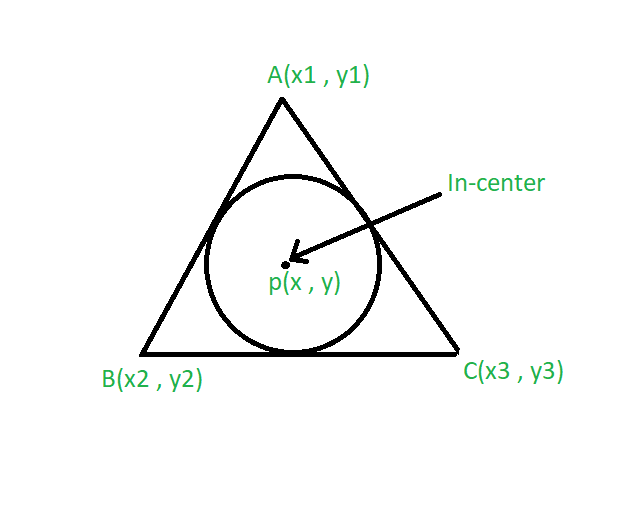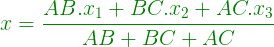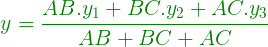GFG App
Open AppBrowser
Continue

# Program to Find the Incenter of a Triangle

Given the vertices of a triangle and length of its sides. A circle is inscribed in a triangle. The task is to find the incenter of a triangle.
Examples:

Input: A(2, 2), B(1, 1), C(3, 1)
Output: (2, 1.5)

Input: A(3, 3), B(1, 2), C(2, 2)
Output: (2.5, 2.83)Approach:

• The center of the circle that touches the sides of a triangle is called its incenter.
• Suppose the vertices of the triangle are A(x1, y1), B(x2, y2) and C(x3, y3).
• Let the side AB = a, BC = b, AC = c then the coordinates of the in-center is given by the formula:••

Below is the implementation of the above approach:

## C++

 `// C++ program to find the` `// incenter of a triangle` `#include ` `using` `namespace` `std;`   `// Driver code` `int` `main()` `{` `    ``// coordinate of the vertices` `    ``float` `x1 = 2, x2 = 1, x3 = 3;` `    ``float` `y1 = 2, y2 = 1, y3 = 1;` `    ``float` `a = 2, b = 1, c = 1;`   `    ``// Formula to calculate in-center` `    ``float` `x = (a * x1 + b *` `                   ``x2 + c * x3) / (a + b + c);` `    ``float` `y = (a * y1 + b * ` `                   ``y2 + c * y3) / (a + b + c);`   `    ``// System.out.print(setprecision(3));` `    ``cout << ``"Incenter = "` `         ``<< ``"("` `<< x << ``", "` `<< y << ``")"``;` `    ``return` `0;` `}`   `// This code is contributed by 29AjayKumar`

## Java

 `// Java program to find the` `// incenter of a triangle`   `import` `java.util.*;` `import` `java.lang.*;`   `class` `GFG {`   `    ``// Driver code` `    ``public` `static` `void` `main(String args[])` `    ``{` `        ``// coordinate of the vertices` `        ``float` `x1 = ``2``, x2 = ``1``, x3 = ``3``;` `        ``float` `y1 = ``2``, y2 = ``1``, y3 = ``1``;` `        ``float` `a = ``2``, b = ``1``, c = ``1``;`   `        ``// Formula to calculate in-center` `        ``float` `x` `            ``= (a * x1 + b * x2 + c * x3) / (a + b + c);` `        ``float` `y` `            ``= (a * y1 + b * y2 + c * y3) / (a + b + c);`   `        ``// System.out.print(setprecision(3));` `        ``System.out.println(``"Incenter= "` `                           ``+ ``"("` `+ x + ``", "` `+ y + ``")"``);` `    ``}` `}`

## Python3

 `# Python3 program to find the` `# incenter of a triangle`   `# Driver code`   `# coordinate of the vertices` `x1 ``=` `2``; x2 ``=` `1``; x3 ``=` `3``;` `y1 ``=` `2``; y2 ``=` `1``; y3 ``=` `1``;` `a ``=` `2``; b ``=` `1``; c ``=` `1``;`   `# Formula to calculate in-center` `x ``=` `(a ``*` `x1 ``+` `b ``*` `x2 ``+` `c ``*` `x3) ``/` `(a ``+` `b ``+` `c);` `y ``=` `(a ``*` `y1 ``+` `b ``*` `y2 ``+` `c ``*` `y3) ``/` `(a ``+` `b ``+` `c);`   `# System.out.print(setprecision(3));` `print``(``"Incenter = ("``, x, ``","``, y, ``")"``);`   `# This code is contributed ` `# by Akanksha Rai`

## C#

 `// C# program to find the` `// incenter of a triangle`   `using` `System;`   `class` `GFG ` `{`   `    ``// Driver code` `    ``public` `static` `void` `Main()` `    ``{` `        ``// coordinate of the vertices` `        ``float` `x1 = 2, x2 = 1, x3 = 3;` `        ``float` `y1 = 2, y2 = 1, y3 = 1;` `        ``float` `a = 2, b = 1, c = 1;`   `        ``// Formula to calculate in-center` `        ``float` `x` `            ``= (a * x1 + b * x2 + c * x3) / (a + b + c);` `        ``float` `y` `            ``= (a * y1 + b * y2 + c * y3) / (a + b + c);`   `        ``// System.out.print(setprecision(3));` `        ``Console.WriteLine(``"Incenter= "` `                        ``+ ``"("` `+ x + ``", "` `+ y + ``")"``);` `    ``}` `}`   `// This code is contributed by vt_m.`

## Javascript

 ``

Output

`Incenter = (2, 1.5)`

Time Complexity: O(1), the code will run in a constant time.
Auxiliary Space: O(1), no extra space is required, so it is a constant.

My Personal Notes arrow_drop_up